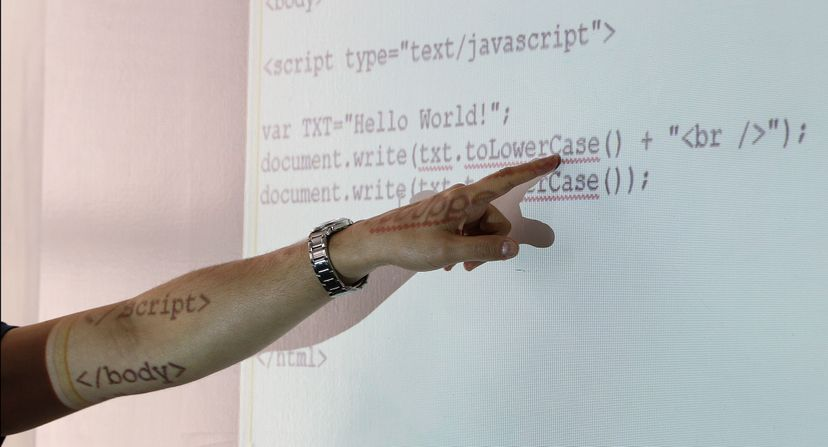# How does JavaScript work and how can I build simple calculators with it?JavaScript is a computer programming language. Pat Greenhouse/The Boston Globe via Getty Images

JavaScript is what is called a Client-side Scripting Language. That means that it is a computer programming language that runs inside an Internet browser (a browser is also known as a Web client because it connects to a Web server to download pages).

The way JavaScript works is interesting. Inside a normal Web page you place some JavaScript code (See How Web Pages Work for details on Web pages). When the browser loads the page, the browser has a built-in interpreter that reads the JavaScript code it finds in the page and runs it.

Web page designers use JavaScript in many different ways. One of the most common is to do field validation in a form. Many Web sites gather information from users in online forms, and JavaScript can help validate entries. For example, the programmer might validate that a person's age entered into a form falls between 1 and 120.

Another way that web page designers use JavaScript is to create calculators. Here are several examples:

To give you an example of an extremely simple JavaScript calculator, the HTML below shows you how to create a Fahrenheit to Celsius converter using JavaScript:

```<html>
<script language="JavaScript">
<!-- hide this script from old browsers
function temp(form)
{
var f = parseFloat(form.DegF.value, 10);
var c = 0;
c = (f - 32.0) * 5.0 / 9.0;
form.DegC.value = c;
}
// done hiding from old browsers -->
</script>
<body>
<FORM>
<h2>Fahrenheit to Celsius Converter</h2>
Enter a temperature in degrees F:
<INPUT NAME="DegF" VALUE="0" MAXLENGTH="15" SIZE=15>
<p>
Click this button to calculate the temperature
in degrees C:
<INPUT NAME="calc" VALUE="Calculate" TYPE=BUTTON
onClick=temp(this.form)>
<p>
Temperature in degrees C is:
</FORM>
</body>
</html>```

If you have read How Web Pages Work and How CGI Scripts Work, then a good portion of this HTML will be familiar. This is the basic structure of any web page:

```<html>
<body>
</body>
</html>```

There is one piece of JavaScript code in the header that is the function to calculate the conversion from Fahrenheit to Celsius:

```<head>
<script>
<!-- hide this script from old browsers
function temp(form)
{
var f = parseFloat(form.DegF.value, 10);
var c = 0;
c = (f - 32.0) * 5.0 / 9.0;
form.DegC.value = c;
}
<!-- done hiding from old browsers -->
</script>

The function is called temp. It contains JavaScript code to calculate a Celsius temperature.

In the body of the page there is a typical form:

```<FORM>
<h2>Fahrenheit to Celsius Converter</h2>
Enter a temperature in degrees F:
<INPUT NAME="DegF" VALUE="0" MAXLENGTH="15" SIZE=15>
<p>
Click this button to calculate the temperature
in degrees C:
<INPUT NAME="calc" VALUE="Calculate" TYPE=BUTTON
onClick=temp(this.form)>
<p>
Temperature in degrees C is:
</FORM>```

This line is key:

```<INPUT NAME="calc" VALUE="Calculate" TYPE=BUTTON
onClick=temp(this.form)>
```

This is a normal button control. When the user clicks it, it calls the function in the head of the page because of the onClick notation.

As programming languages go, JavaScript is average difficulty. It is not especially hard to learn how to use it if you already understand programming, but if you are new to programming it is certainly not an easy language to start with. What you can do, however, is modify this sample code and expand it to create other calculators.